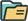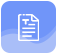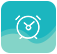# 2021自考考试高等数学（一）基础练习3

### 来源：成人学历报考中心发布日期：2022-04-22

1、微分方程y'=1的通解为( )

A y=x

B y=Cx

C y=C-x

D y=C+x

答案：D

2、微分方程y'-4y=0的特征根为( )

A 0，4

B-2，2

C-2，4

D 2，4

答案：B

3、微分方程y′-y=0的通解为( ).

A y=ex+C

B y=e-x+C

C y=Cex

D y=Ce-x

答案：C

4、下列命题中正确的为( )

A若xo为f(x)的极值点，则必有，f'(xo)=0

B若f'(xo)=0，则点xo必为f(x)的极值点

C若f'(xo)≠0，则点xo必定不为f(x)的极值点

D若f(x)在点xo处可导，且点xo为f(x)的极值点，则必有f'(xo)=0

答案：D

5、当x→0时，kx是sinx的等价无穷小量，则k等于( ).

A 0

B 1

C 2

D 3

答案：B

6、当x→0时，2x+x2是x的( )

A等价无穷小

B较低阶无穷小

C较高阶无穷小

D同阶但不等价的无穷小

答案：D

7、微分方程(y′)2=x的阶数为( ).

A 1

B 2

C 3

D 4

答案：A

8、对于微分方程y'-2y'+y=xex，利用待定系数法求其特解y*时，下列特解设法正确的是( )A.Y*=(Ax+B)ex B.y*=x(Ax+

A ex

B y*=Ax3ex

C Y*=x2(Ax+

D ex

答案：D

9、用待定系数法求微分方程Y'-y=xex的一个特解时，特解的形式是(式中a、b是常数)( )

A(ax2+bx)ex

B(a,x2+b)ex

C ax2ex

D(ax+6)ex

答案：A

10、方程x+y-z=0表示的图形为( ).

A旋转抛物面

B平面

C锥面

D椭球面

答案：B

## 声明：

（一）由于考试政策等各方面情况的不断调整与变化，本网站所提供的考试信息仅供参考，请以权威部门公布的正式信息为准。

（二）本网站在文章内容来源出处标注为其他平台的稿件均为转载稿，免费转载出于非商业性学习目的，版权归原作者所有。如您对内容、版权等问题存在异议请与本站联系，我们会及时进行处理解决。题库资料
•真题精炼
依据历年真题，快速梳理
知识点，检验学习效果
点击查看
•模拟试题
依据考试大纲高质量
模拟试卷
点击查看
•教材大纲
全力冲刺，紧抓考前机会
全力冲考
点击查看